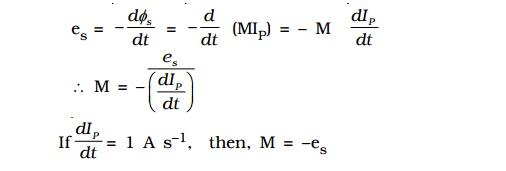Home | | Physics | Mutual induction and Coefficient of mutual induction

# Mutual induction and Coefficient of mutual induction

Whenever there is a change in the magnetic flux linked with a coil, there is also a change of flux linked with the neighbouring coil, producing an induced emf in the second coil.

## Mutual induction

Whenever there is a change in the magnetic flux linked with a coil, there is also a change of flux linked with the neighbouring coil, producing an induced emf in the second coil. This phenomenon of producing an induced emf in a coil due to the change in current in the other coil is known as mutual induction.P and S are two coils placed close to each other (Fig. 4.6). P is connected to a battery through a key K. S is connected to a galvanometer G. On pressing K, current in P starts increasing from zero to a maximum value. As the flow of current increases, the magnetic flux linked with P increases. Therefore, magnetic flux linked with S also increases producing an induced emf in S. Now, the galvanometer shows the deflection. According to Lenz’s law the induced current in S would oppose the increase in current in P by flowing in a direction opposite to the current in P, thus delaying the growth of current to the maximum value. When the key ‘K’ is released, current starts decreasing from maximum to zero value, consequently magnetic flux linked with P decreases. Therefore magnetic flux linked with S also decreases and hence, an emf is induced in S. According to Lenz’s law, the induced current in S flows in such a direction so as to oppose the decrease in current in P thus prolonging the decay of current.

## Coefficient of mutual induction

IP is the current in coil P and φs is the magnetic flux linked with coil S due to the current in coil P.where M is a constant of proportionality and is called the coefficient of mutual induction or mutual inductance between the two coils.

IfIP = 1A, then, M = φs

Thus, coefficient of mutual induction of two coils is numerically equal to the magnetic flux linked with one coil when unit current flows through the neighbouring coil. If es is the induced emf in the coil (S) at any instant of time, then from the laws of electromagnetic induction,Thus, the coefficient of mutual induction of two coils is numerically equal to the emf induced in one coil when the rate of change of current through the other coil is unity. The unit of coefficient of mutual induction is henry.

One henry is defined as the coefficient of mutual induction between a pair of coils when a change of current of one ampere per second in one coil produces an induced emf of one volt in the other coil.

The coefficient of mutual induction between a pair of coils depends on the following factors

i.            Size and shape of the coils, number of turns and permeability of material on which the coils are wound.

ii.            proximity of the coils

Two coils P and S have their axes perpendicular to each other (Fig. 4.7a). When a current is passed through coil P, the magnetic flux linked with S is small and hence, the coefficient of mutual induction between the two coils is small.

The two coils are placed in such a way that they have a common axis (Fig. 4.7b). When current is passed through the coil P the magnetic flux linked with coil S is large and hence, the coefficient of mutual induction between the two coils is large.If the two coils are wound on a soft iron core (Fig 4.7c) the mutual induction is very large.

## Mutual induction of two long solenoids.

S1 and S2 are two long solenoids each of length l. The solenoid S2 is wound closely over the solenoid S1 (Fig 4.8).N1 and N2 are the number of turns in the solenoids S1 and S2 respectively. Both the solenoids are considered to have the same area of cross section A as they are closely wound together. I1 is the current flowing through the solenoid S1. The magnetic field B1 produced at any point inside the solenoid S1 due to the current I1 isThe magnetic flux linked with each turn of S2 is equal to B1A.

Total magnetic flux linked with solenoid S2 having N2 turns iswhere M is the coefficient of mutual induction between S1 and S2.

From equations (2) and (3)Study Material, Lecturing Notes, Assignment, Reference, Wiki description explanation, brief detail
Physics : Effects of electric current : Higher Secondary(12 Std) : Mutual induction and Coefficient of mutual induction |